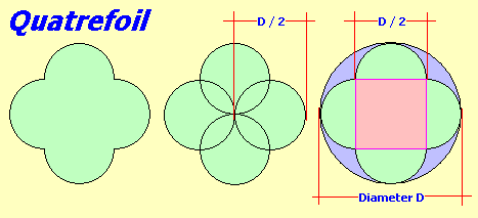# Calculate the Area and Perimeter of a Quatrefoil

This calculator will work for a quatrefoil shape where the diameter of the inscribed circles are equal to the radius of the circumscribed circle.### Circumscribed Circle Formula

R = D ÷ 2
where R = radius, D = diameter

Area
A = π * D² ÷ 4
where A = area, π = 3.14159..., D = diameter

Circumference
C = 2 * π * D ÷ 2
where C = circumference, π = 3.14159...., D = diameter

### Quatrefoil Formula

Area
A =(s * s) + 2 (π * r² ÷ 4)
where a = area, s = D/2, r = D/2

Perimeter
P = (2 * π * D ÷ 2) * 2

### Quartrefoil

Select unit:
Diameter of circle {{selectedunit.m1}}
decimal rounding # of digits in results

#### Results:

 Radius {{selectedunit.m1}} Area of circle {{selectedunit.m1}}² Circumference of circle {{selectedunit.m1}} Area of quartrefoil {{selectedunit.m1}}² Perimeter of quartrefoil {{selectedunit.m1}}

#### Calculation

1. enter the diameter of the circumscribed circle
2. number of decimal places required in results

#### Results

1. area of circumscribed circle
2. circumference
3. area of quatrefoil
4. perimeter of quartrefoil